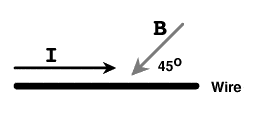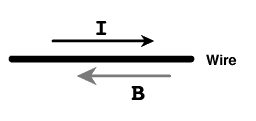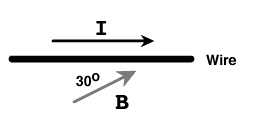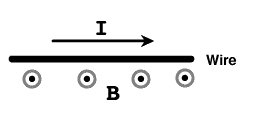# Direction of magnetic force

## Homework Statement

Decide from the following list of possibilities what is the appropriate direction of the force on the wire for each of the diagrams 1 through 4:
A up
B down
C left
D right
E into the page
F out of the page
G None of these
H No force

Diagram 1Diagram 2Diagram 3Diagram 4F = IL x B

## The Attempt at a Solution

I tried using the right hand rule for this. Is it correct that the fingers point in the same direction as the current, thumb in the direction of B and F is the direction the fingers curl? For diagram 1 and 3, I don't get how I'm supposed to position my hand. For diagram 2, I said there is no force because I and B are parallel and going in opposite directions. For diagram 4, is B pointing out of the page? I said that F is up but I'm not 100% sure.

The right-hand rule I use is as follows:

For $$\vec A \times \vec B = \vec C$$
Lay the side of your palm on the table for A, curl your fingers in the direction of B, and your thumb will point in the direction of C.

For the case of $$\frac{\vec F}{I}=\vec \ell \times \vec B$$
The side of your palm is in the direction of the current, your fingers curl in the direction of the B-field, and the force is given by the direction of the thumb.

For the magnetic field produced by a current carrying wire (This question does not refer to this at all! So don't get confused., point your thumb in the direction of the current, and your fingers will curl along the B-field lines.

This image below should help clear things up. It gives three examples. The first is for determining the magnetic field in the vicinity of a current-carrying wire, the second for the force on a current-carrying wire in an external field and the third for a free particle moving under the influence of an external field (Note that here charge matters!)
http://www.uwsp.edu/physastr/kmenning/images/gc6.20.T1.gif [Broken]

Last edited by a moderator:
That sort of helps so is this how it goes?
diagram 1 = out of the page
diagram 2 = down
diagram 3 = up
diagram 4 = right

No, none of those are correct. Please show your work and we'll see where you went wrong so we know how to avoid that mistake again. :)

For each one, I used the right hand rule. I placed the side of my palm in the direction of I, then I curled my fingers in the direction of B and then the direction my thumb was in was F. I was probably wrong on all of them because I just don't know how my fingers are supposed to curl at the same angle B makes with the wire (like in 1 and 3). And I'm still not totally sure what direction B is in diagram 4.

Nevermind, I got it. It goes EHFB. My hand just wasn't working right.